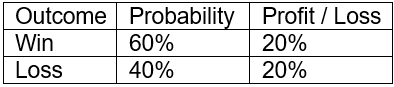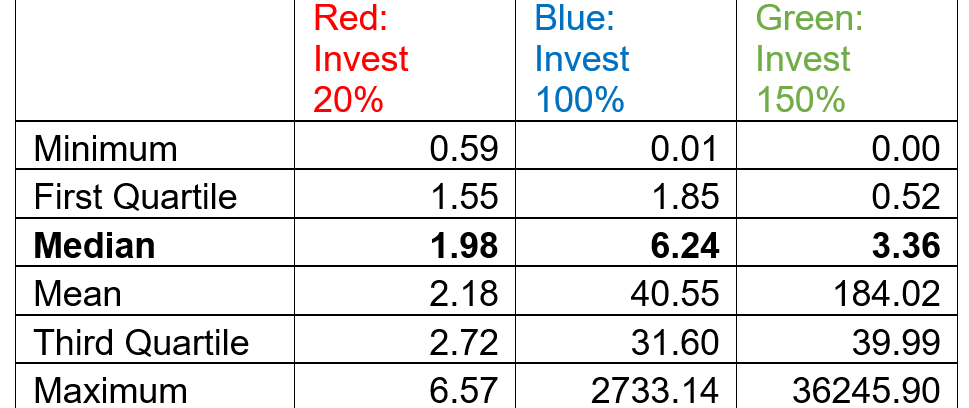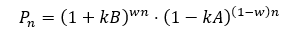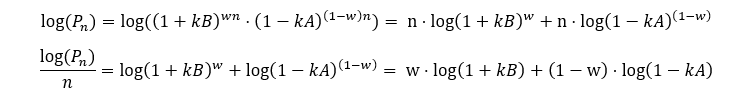# The Kelly Criterion: You Don’t Know the Half of ItDespite expending substantial resources on a formal financial education, I did not encounter the Kelly criterion in business school or the CFA curriculum. I came across it almost by accident, in William Poundstone’s delightful book Fortune’s Formula.

Created in 1956 by John Kelly, a Bell Labs scientist, the Kelly criterion is a formula for sizing bets or investments from which the investor expects a positive return. It is the only formula I’ve seen that comes with a mathematical proof explaining why it can deliver higher long-term returns than any alternative.

In my view, the formula is consistent with the value investing concept of a margin of safety and leads to concentrated portfolios in which the dominant ideas have the greatest edge and smallest downside.Despite its relative obscurity and lack of mainstream academic support, the Kelly criterion has attracted some of the best-known investors on the planet, Warren Buffett, Charlie Munger, Mohnish Pabrai, and Bill Gross, among them. While the Kelly formula requires an estimate of the probability distribution of investment outcomes ahead of time, i.e., a crystal ball, its mainstream alternative, Harry Markowitz’s mean/variance optimization, calls for an estimate of the covariance matrix, which for a bottom-up investor, I believe is much more difficult to obtain.

After reading Poundstone’s book, I wanted to apply the Kelly criterion to my own investing. I learn by example and my math is rusty, so I looked for a short, non-technical article about how the formula can work in an equity-like investment.

Unfortunately, most of the sources I found use the wrong formula.

The top article in a Google search for “Kelly calculator equity” presents a simple, stylized investment with a 60% chance of gaining and a 40% chance of losing 20% in each simulation. No other outcomes are possible, and the investment can be repeated across many simulations, or periods.It’s clearly a good investment, with a positive expectation: E(x) = 60% * 20 + 40% * (-20%) = 4%. But what share of the portfolio should it take up? Too small an allocation and the portfolio will lose out on growth. Too large and a few unlucky outcomes — even a single one — could depress it beyond recovery or wipe it out altogether. So what percentage allocation, consistently applied, maximizes the portfolio’s potential long-term growth rate?

The article I found and many like it use the formula Kelly % = W – [(1 – W) / R], where W is the win probability and R is the ratio between profit and loss in the scenario.

For this investment, W is 60% and R is 1 (20%/20%). The loss is expressed as a positive. Plugging in the numbers, the Kelly % = 60% – [(1 – 60%) / (20%/20%)] = 20%. In other words, a 20% allocation to the investment maximizes the portfolio’s potential long-term growth.

This is simply incorrect. The error is intuitive, empirical, and mathematical. The formula does not account for the magnitude of potential profits and losses (volatility), only their ratio to each other. Indeed, the article does not even list the potential gain or loss. Change the potential profit and loss from 20% each to 200% each, and the investment becomes 10 times more volatile. Yet the ratio R stays the same — 200%/200% = 1 — as does the formula’s resulting 20% optimal allocation.Consider a simulation with three different allocation scenarios, all replicating the same investment over and over: Red allocates 20% of the portfolio, as the articles suggests, Blue goes all in at 100%, and Green levers up to 150%. The chart below visualizes how the simulation plays out after 100 rounds.In the Red, “Kelly optimal” scenario, a 20% allocation earned a relatively puny 2x return. The Blue, all-in option generated a 6.2x return. Green outpaced Blue for a time but a string of losses in the later rounds led to a 3.4x return.

This wasn’t just a lucky outcome for Blue. Run the simulation 1,000 times and Blue beats Red 79% and Green 67% of the time. Blue’s median return was at least 3x better than Red’s and almost 2x better than Green’s. In short, the 20% allocation is too conservative and the Green option too aggressive.

Ending Portfolio Value after 1,000 Simulations (In Dollars, Starting with \$1 in Period 1)The Kelly formula in the first scenario — Kelly % = W – [(1 – W)/R] — is not an anomaly. It turns up in many other sources, including NASDAQMorningstar, Wiley’s For Dummies series, Old School Value, etc., and is analogous to the one in Fortune’s FormulaKelly % = edge/odds.

But the formula works only for binary bets where the downside scenario is a total loss of capital, as in -100%. Such an outcome may apply to blackjack and horse racing, but rarely to capital markets investments.

If the downside-case loss is less than 100%, as in the scenario above, a different Kelly formula is required: Kelly % = W/A – (1 – W)/B, where W is the win probability, B is the profit in the event of a win (20%), and A is the potential loss (also 20%).

Plugging in the values for our scenario: Kelly % = 60%/20% – (1 – 60%)/20% = 100%, which was Blue’s winning allocation.

The theoretical downside for all capital market investments is -100%. Bad things happen. Companies go bankrupt. Bonds default and are sometimes wiped out. Fair enough.

But for an analysis of the securities in the binary framework implied by the edge/odds formula, the downside-scenario probability must be set to the probability of a total capital loss, not the much larger probability of some loss.

There are many criticisms of the Kelly criterion. And while most are beyond the scope of this article, one is worth addressing. A switch to the “correct” Kelly formula — Kelly % = W/A – (1 – W)/B — often leads to significantly higher allocations than the more popular version.

Most investors won’t tolerate the volatility and resulting drawdowns and will opt to reduce the allocation. That’s well and good — both variations of the formula can be scaled down — but the “correct” version is still superior. Why? Because it explicitly accounts for and encourages investors to think through the downside scenario.

And in my experience, a little extra time spent thinking about that is richly rewarded.#### Appendix: Supporting Math

Here is a derivation of the Kelly formula: An investor begins with \$1 and invests a fraction (k) of the portfolio in an investment with two potential outcomes. If the investment succeeds, it returns B and the portfolio will be worth 1 + kB. If it fails, it loses A and the portfolio will be worth 1 – kA.

The investment’s probability of success is w. The investor can repeat the investment as often as desired but must invest the same fraction (k) each time. What fraction k will maximize the portfolio in the long term?

In the long term, after n times where n is large, the investor is expected to have w * n wins and (1 – w)n losses. The portfolio P will be worth:We would like to solve for the optimal k:To maximize , we take its derivative with respect to k and set it to 0:Solving for k:Note that if the downside-scenario loss is total (A = 1), this formula simplifies to the more popular version quoted above because R = B/A = B/1 = B, so:#### Appendix: Supporting Code

Below is the R code used to produce the simulation and the charts above.

##########################################################

#Kelly Simulation, Binary Security

# by Alon Bochman

##########################################################

trials = 1000 # Repeat the simulation this many times

periods = 100 # Periods per simulation

winprob = 0.6 # Win probability per period

returns = c(0.2,-0.2) # Profit if win, loss if lose

fractions = c(0.2,1,1.5) # Competing allocations to test

library(ggplot2)

library(reshape2)

library(ggrepel)

percent <- function(x, digits = 2, format = “f”, …) {

paste0(formatC(100 * x, format = format, digits = digits, …), “%”)

}

set.seed(136)

wealth = array(data=0,dim=c(trials,length(fractions),periods))

wealth[,,1] =1 #Eq=1 in period 1

#Simulation loop

for(trial in 1:trials) {

outcome = rbinom(n=periods, size=1, prob=winprob)

ret = ifelse(outcome,returns,returns)

for(i in 2:length(ret)) {

for(j in 1:length(fractions)) {

bet = fractions[j]

wealth[trial,j,i] = wealth[trial,j,i-1] * (1 + bet * ret[i])

}

}

}

#Trial 1 Results

view.trial = 1

d <- melt(wealth)

colnames(d) = c(‘Trial’,’Fraction’,’Period’,’Eq’)

d = subset(d,Trial ==view.trial)

d\$Fraction = as.factor(d\$Fraction)

levels(d\$Fraction) = paste(“Invest “,percent(fractions,digits=0),sep=”)

d[d\$Period == periods,’Label’] = d[d\$Period == periods,’Eq’]

ggplot(d, aes(x=Period,y=Eq, col=Fraction)) +

geom_line(size=1) + scale_y_log10() +

labs(y=”Portfolio Value”,x=”Period”) +

guides(col=guide_legend(title=”Allocation”)) +

theme(legend.position = c(0.1, 0.9)) +

scale_color_manual(values=c(“red”, “blue”,”green”)) + #Adjust if >2 allocations

geom_label_repel(aes(label = round(Label, digits = 2)),

nudge_x = 1, show.legend = F, na.rm = TRUE)

#All-Trial Results

d = data.frame(wealth[,,periods]) #Last period only

colnames(d) = paste(“Invest “,percent(fractions,digits=0),sep=”)

summary(d)

nrow(subset(d,d[,2] > d[,1])) / trials #Blue ahead of red

nrow(subset(d,d[,2] > d[,3])) / trials #Blue ahead of green

If you liked this post, don’t forget to subscribe to the Enterprising Investor.

All posts are the opinion of the author. As such, they should not be construed as investment advice, nor do the opinions expressed necessarily reflect the views of CFA Institute or the author’s employer.

Image credit: ©Getty Images/ PATCHARIN SIMALHEK

##### Alon Bochman, CFA

Alon Bochman, CFA, is a partner in Genpact’s (NYSE: G) capital markets consulting practice, based in New York. He works with asset managers and banks to help them make better decisions with data. Previously, he spent two years managing an equity portfolio for SC Fundamental. Bochman began his career as a programmer by co-founding a social networking software firm eventually acquired by Thomson-Reuters. He holds an MBA from Columbia Business School and a BA from the University at Albany.